2022: SklogWiki celebrates 15 years on-line

# Gibbs energy function

Definition: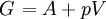$\left.G\right.=A+pV$

where p is the pressure, V is the volume, and A is the Helmholtz energy function, i.e.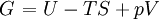$\left.G\right.=U-TS+pV$

Taking the total derivative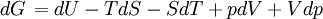$\left.dG\right.=dU-TdS-SdT+pdV+Vdp$

From the Second law of thermodynamics one obtains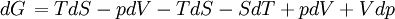$\left.dG\right.=TdS -pdV-TdS-SdT+pdV+Vdp$

thus one arrives at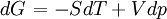$\left.dG\right.=-SdT+Vdp$

For G(T,p) we have the following total differential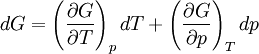$dG=\left(\frac{\partial G}{\partial T}\right)_p dT + \left(\frac{\partial G}{\partial p}\right)_T dp$